Courses

# Design For Static Loading (Part - 2) Mechanical Engineering Notes | EduRev

## Mechanical Engineering : Design For Static Loading (Part - 2) Mechanical Engineering Notes | EduRev

The document Design For Static Loading (Part - 2) Mechanical Engineering Notes | EduRev is a part of the Mechanical Engineering Course Machine Design.
All you need of Mechanical Engineering at this link: Mechanical Engineering

Yield criteria

There are numerous yield criteria, going as far back as Coulomb (1773). Many of these were originally developed for brittle materials but were later applied to ductile materials. Some of the more common ones will be discussed briefly here.

Maximum principal stress theory

( Rankine theory) According to this, if one of the principal stresses σ1 (maximum principal stress), σ2 (minimum principal stress) or σ3 exceeds the yield stress, yielding would occur. In a two dimensional loading situation for a ductile material where tensile and compressive yield stress are nearly of same magnitude

σ1 = ± σ
σ2 = ±σy

Using this, a yield surface may be drawn, as shown in figure- 3.1.4.1.1. Yielding occurs when the state of stress is at the boundary of the rectangle. Consider, for example, the state of stress of a thin walled pressure vessel. Here σ1= 2σ2, σ1 being the circumferential or hoop stress and σthe axial stress. As the pressure in the vessel increases the stress follows the dotted line. At a point (say) a, the stresses are still within the elastic limit but at b, σ1 reaches σy although σ2 is still less than σy. Yielding will then begin at point b. This theory of yielding has very poor agreement with experiment. However, the theory has been used σ2 successfully for brittle materials.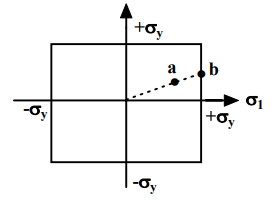3.1.4.1.1F- Yield surface corresponding to maximum principal stress theory

3.1.4.2 Maximum principal strain theory (St. Venant’s theory)

According to this theory, yielding will occur when the maximum principal strain just exceeds the strain at the tensile yield point in either simple tension or compression. If ε1 and ε2 are maximum and minimum principal strains corresponding to σ1 and σ2, in the limiting case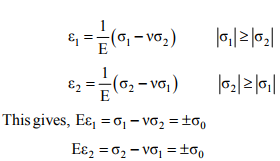The boundary of a yield surface in this case is thus given as shown in figure3.1.4.2.1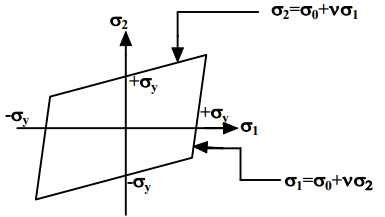3.1.4.2.1- Yield surface corresponding to maximum principal strain theory

Maximum shear stress theory ( Tresca theory)

According to this theory, yielding would occur when the maximum shear stress just exceeds the shear stress at the tensile yield point. At the tensile yield point σ2= σ3 = 0 and thus maximum shear stress is σy/2. This gives us six conditions for a three-dimensional stress situation: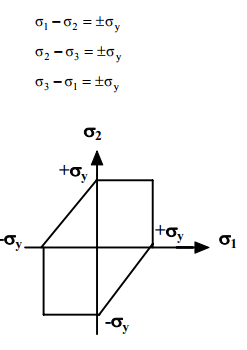3.1.4.3.1F- Yield surface corresponding to maximum shear stress theory

In a biaxial stress situation ( figure-3.1.4.3.1) case, σ3 = 0 and this gives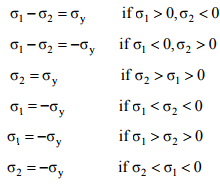This criterion agrees well with experiment. In the case of pure shear, σ1 = - σ2 = k (say), σ3 = 0 and this gives σ1- σ2 = 2k= σy This indicates that yield stress in pure shear is half the tensile yield stress and this is also seen in the Mohr’s circle ( figure- 3.1.4.3.2) for pure shear.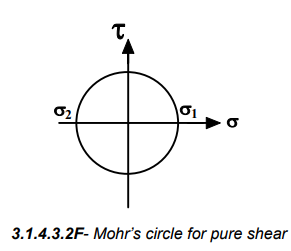Maximum strain energy theory ( Beltrami’s theory)

According to this theory failure would occur when the total strain energy absorbed at a point per unit volume exceeds the strain energy absorbed per unit volume at the tensile yield point. This may be given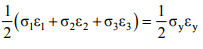by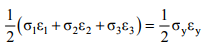Substituting, ε1, ε2 , εand εy in terms of stresses we have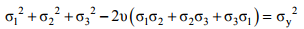This may be written as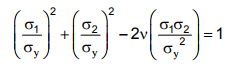This is the equation of an ellipse and the yield surface is shown in figure3.1.4.4.1 .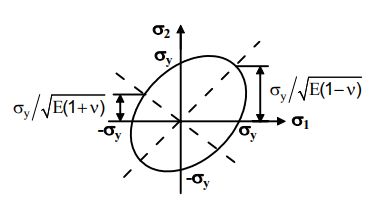3.1.4.4.1F- Yield surface corresponding to Maximum strain energy theory.

It has been shown earlier that only distortion energy can cause yielding but in the above expression at sufficiently high hydrostatic pressure σ1 = σ2 = σ3 = σ (say), yielding may also occur. From the above we may write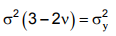and if ν ~ 0.3, at stress level lower than yield stress, yielding would occur. This is in contrast to the experimental as well as analytical conclusion and the theory is not appropriate.

Distortion energy theory( von Mises yield criterion)

According to this theory yielding would occur when total distortion energy absorbed per unit volume due to applied loads exceeds the distortion energy absorbed per unit volume at the tensile yield point. Total strain energy ET and strain energy for volume change EV can be given as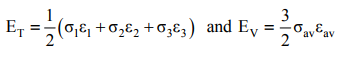Substituting strains in terms of stresses the distortion energy can be given as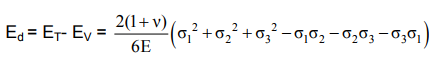At the tensile yield point, σ1 = σy , σ23 = 0 which gives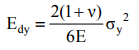The failure criterion is thus obtained by equating Ed and Edy , which gives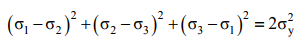In a 2-D situation if σ3 = 0, the criterion reduces to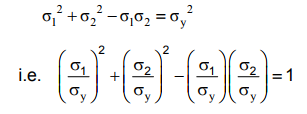This is an equation of ellipse and the yield surface is shown in figure-3.1.4.5.1 . This theory agrees very well with experimental results and is widely used for ductile materials.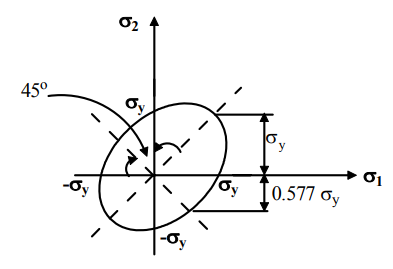3.1.4.5.1F- Yield surface corresponding to von Mises yield criterion

Superposition of yield surface

A comparison among the different failure theories can be made by superposing the yield surfaces as shown in figure- 3.1.5.1.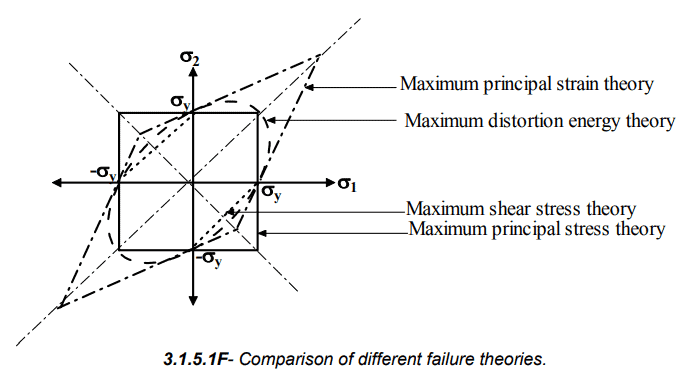It is clear that an immediate assessment of failure probability can be made just by plotting any experimental in the combined yield surface. Failure of ductile materials is most accurately governed by the distortion energy theory where as the maximum principal strain theory is used for brittle materials.

Offer running on EduRev: Apply code STAYHOME200 to get INR 200 off on our premium plan EduRev Infinity!

## Machine Design

57 videos|71 docs|102 tests

,

,

,

,

,

,

,

,

,

,

,

,

,

,

,

,

,

,

,

,

,

;